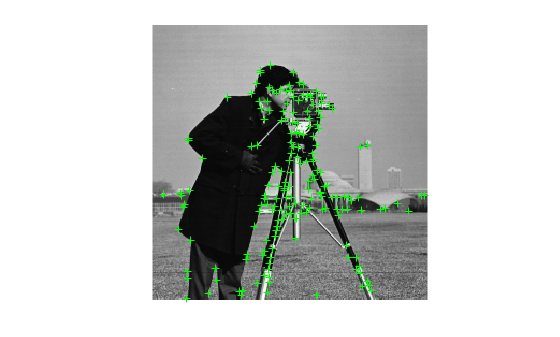# size

Return size of points object

## Syntax

``sz = size(points)``
``sz = size(points,1)``
``sz = size(points,dimension)``
``[M,N] = size(points)``

## Description

example

````sz = size(points)` returns the size of the points object. `sz` is defined as the vector [`length`(`points`),`1`].```
````sz = size(points,1)` returns the length of `points`.```
````sz = size(points,dimension)` returns the length of the points in the specified dimension.```
````[M,N] = size(points)` returns `length`(`points`) for M and 1 for N```

## Examples

collapse all

`I = imread('cameraman.tif');`

Detect corner features.

`featurePoints = detectHarrisFeatures(I);`

Find the size of the feature points object.

`sz = size(featurePoints)`
```sz = 1×2 184 1 ```

Plot feature image with detected features.

```imshow(I); hold on; plot(featurePoints);```## Input Arguments

collapse all

Points object,specified as one of the point feature objects described in Point Feature Types. The object contains information about the feature points detected in the input image. To obtain points, use the appropriate detect function that pairs with the point feature type.

Dimension, returned as an integer. For `dim` >= 2, the object returns `1`.

## Version History

Introduced in R2012a